Homework Help Question & Answers

# Use the electron arrangement interactive to complete the table. Sublevel Number of electrons needed to fill...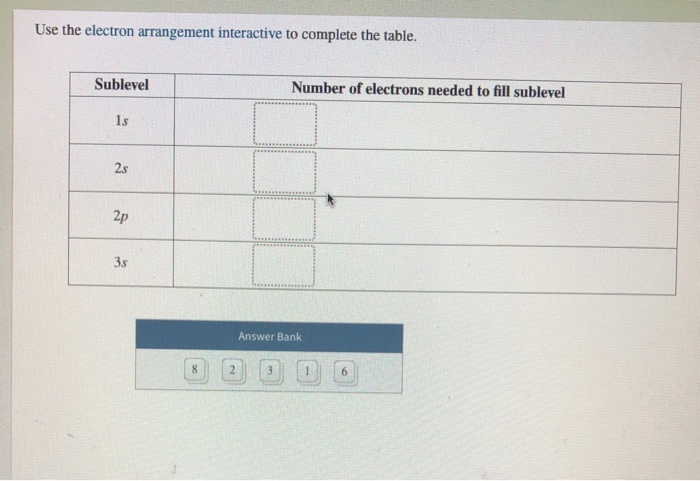Use the electron arrangement interactive to complete the table. Sublevel Number of electrons needed to fill sublevel Answer Bank

#### Homework Answers

Answer #1 ✔ Recommended Answer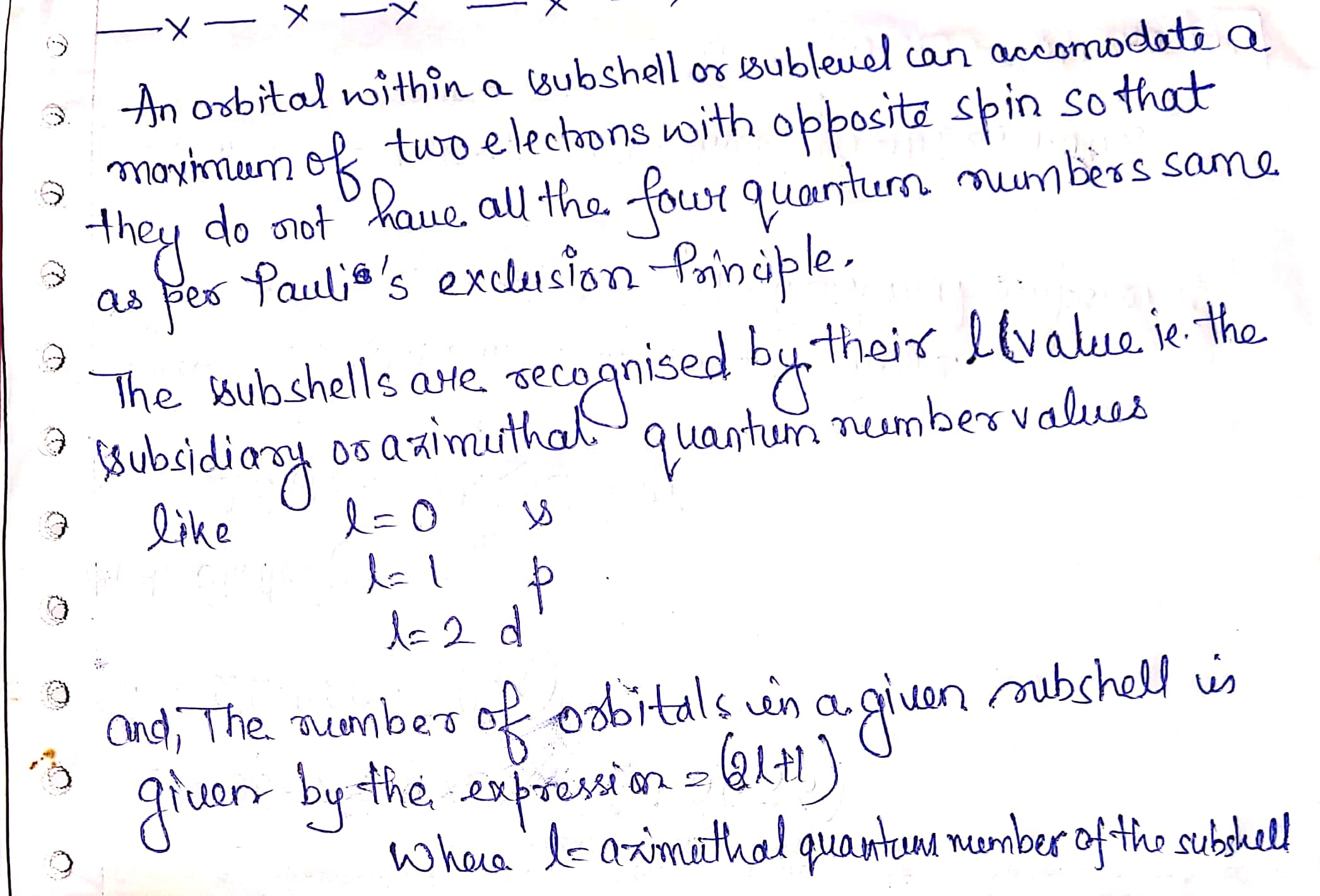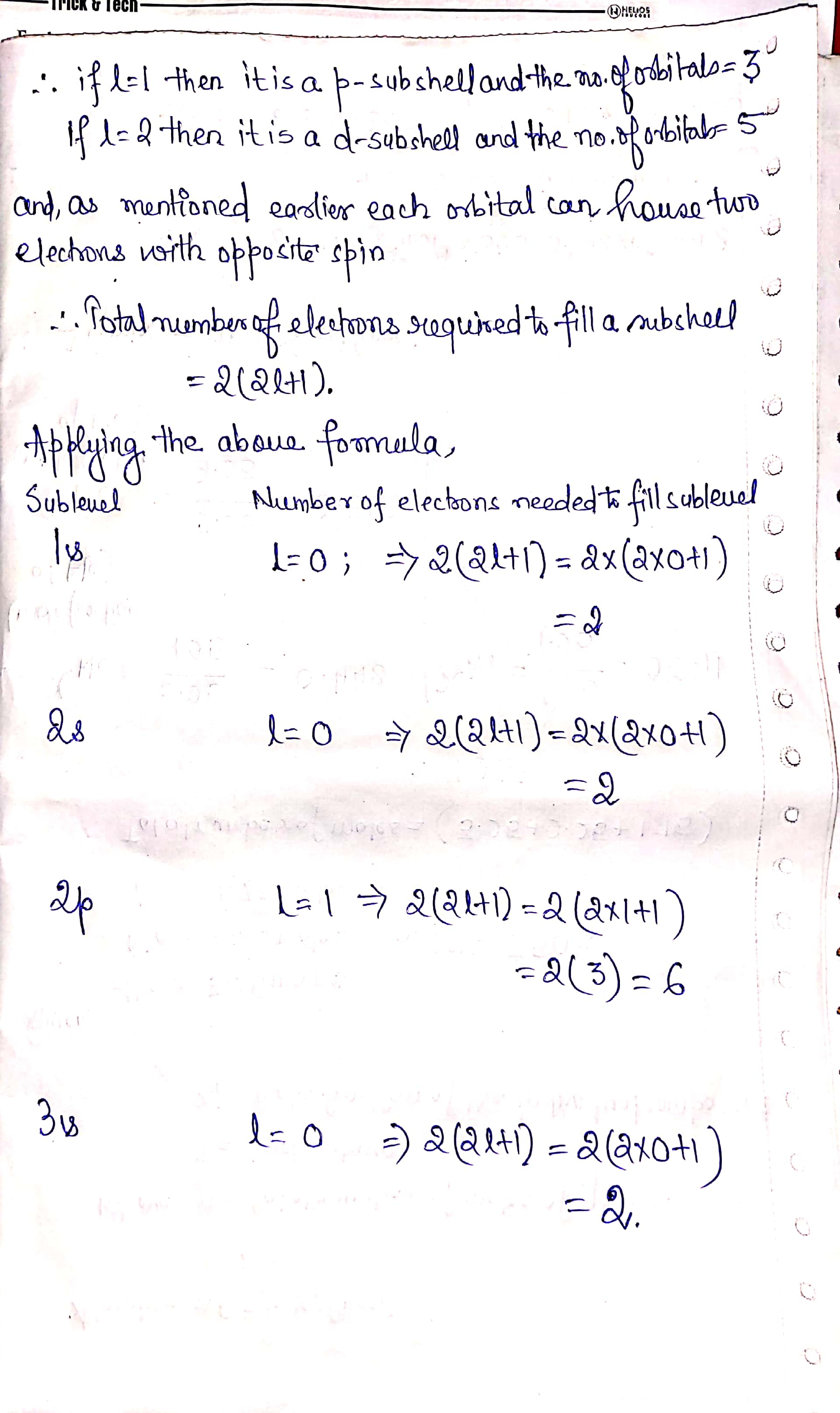Add a comment
Know the answer?
Your Answer:

#### Post as a guest

Your Name:

What's your source?

#### Earn Coin

Coins can be redeemed for fabulous gifts.

Similar Homework Help Questions
• ### Use the electron arrangement interactive to determine the order in which the orbitals are filled, from...

Use the electron arrangement interactive to determine the order in which the orbitals are filled, from filled first to filled last 3px,3py,3pz 1s 2px,2py,2pz 3s 2s

• ### Fill in the missing information about the atoms in the table below. number of electrons number...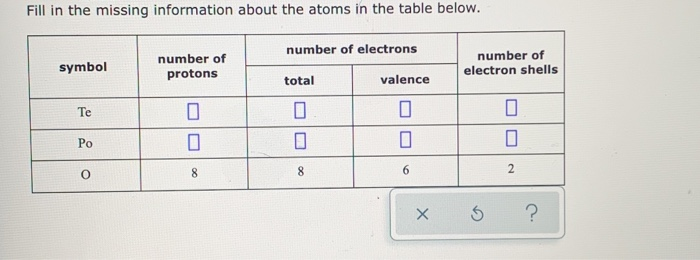Fill in the missing information about the atoms in the table below. number of electrons number of electron shells number of symbol protons valence total Te Po 2 6 8 8 O X

• ### Fill in the information missing from this table: Some electron subshells subshell principal quantum number n...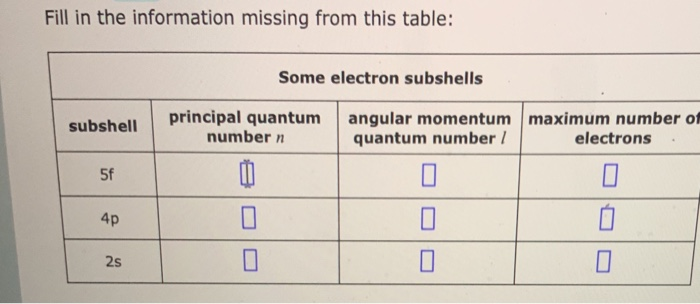Fill in the information missing from this table: Some electron subshells subshell principal quantum number n angular momentum maximum number of quantum number / electrons. 0

• ### Question 18 of 21 100/100 Use the spectroscopy interactive to complete the table with the corresponding lines for e...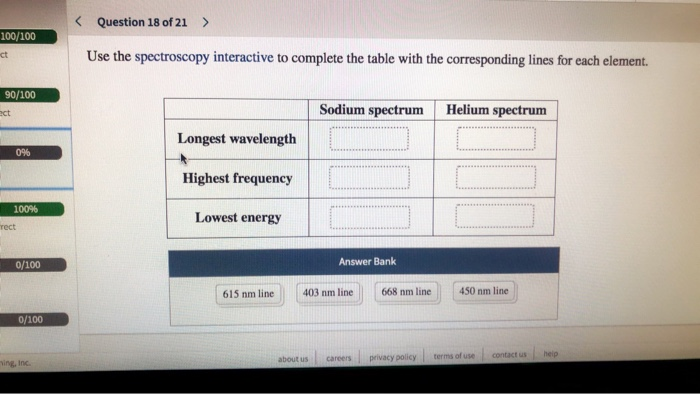Question 18 of 21 100/100 Use the spectroscopy interactive to complete the table with the corresponding lines for each element. ct 90/100 Sodium spectrum Helium spectrum ect Longest wavelength 0% Highest frequency 100% Lowest energy rect Answer Bank 0/100 403 nm line 668 nm line 450 nm line 615 nm line 0/100 contact us heip terms of use privacy policy about us careers ing, Inc. Question 18 of 21 100/100 Use the spectroscopy interactive to complete the table with the...

• ### How many electrons in an atom can have each of the following quantum number or sublevel...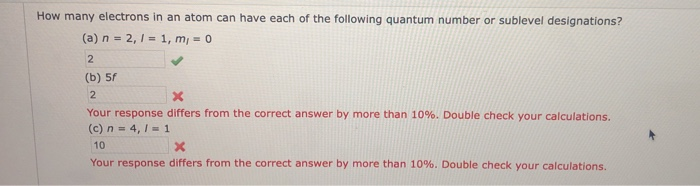How many electrons in an atom can have each of the following quantum number or sublevel designations? (a) n = 2, 1 = 1, m = 0 (b) 5f Your response differs from the correct answer by more than 10%. Double check your calculations, (c) n = 4,1 = 1 10 Your response differs from the correct answer by more than 10%. Double check your calculations.

• ### 13. Write the complete electron configuration and indicate the number of unpaired electrons for each of the followin...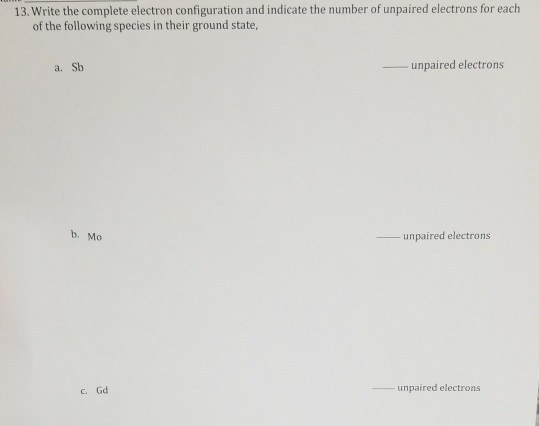13. Write the complete electron configuration and indicate the number of unpaired electrons for each of the following species in their ground state, a. Sb - unpaired electrons b. Mo - unpaired electrons CGd unpaired electrons

• ### Fill in the ....ormation missing from this table: Some electron subshells xs ? subshell principal quantum...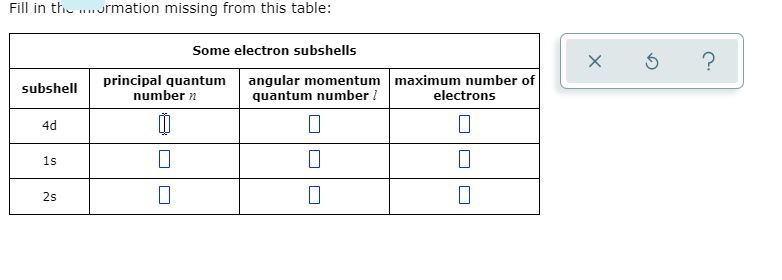Fill in the ....ormation missing from this table: Some electron subshells xs ? subshell principal quantum number n angular momentum maximum number of quantum number / electrons 4d 25

• ### 4 (a) Complete the table to show the number of electrons and number of neutrons (2)...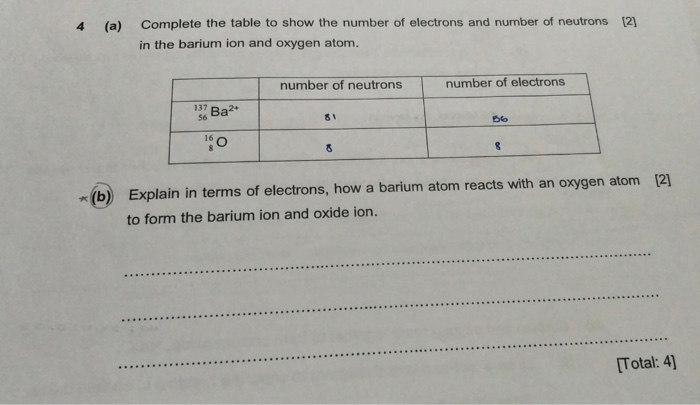4 (a) Complete the table to show the number of electrons and number of neutrons (2) in the barium ion and oxygen atom. number of neutrons number of electrons 137 Baa* 56 81 be 10 8 8 *(b) Explain in terms of electrons, how a barium atom reacts with an oxygen atom  to form the barium ion and oxide ion. [Total: 4) 2.6 Кеу electrons of atom X electrons of atom Y Ryan drew a 'dot-and-cross' diagram to show...

• ### 2.- Which equation shows quantized change in energy? 3.- What is the max number of electrons...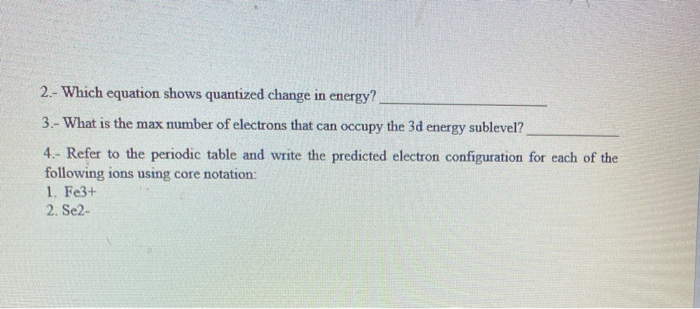2.- Which equation shows quantized change in energy? 3.- What is the max number of electrons that can occupy the 3d energy sublevel? 4.- Refer to the periodic table and write the predicted electron configuration for each of the following ions using core notation: 1. Fe3+ 2. Se2-

• ### please help me to fill this table in details Table 7.1-(Complete and submit to your instructor) Formula Number of Le...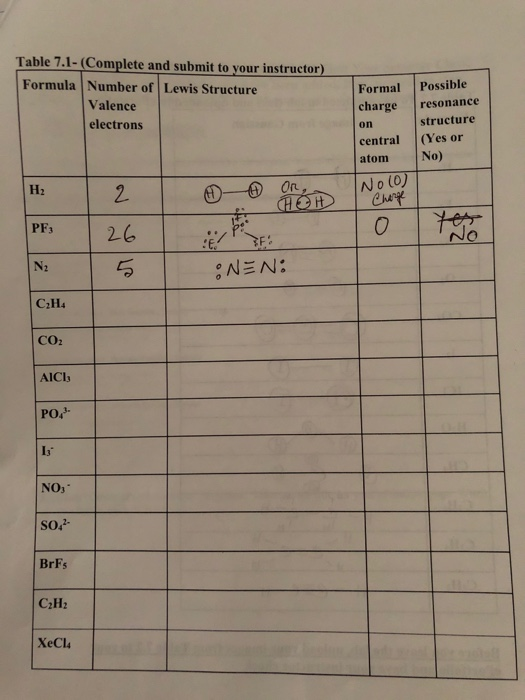please help me to fill this table in details Table 7.1-(Complete and submit to your instructor) Formula Number of Lewis Structure Valence electrons Formal charge on central atom Possible resonance structure (Yes or No) Hz 2 No (0) Chery PF3 26 N2 ENEN: C2H4 CO2 AICI: PO/ NO, SO- BrFs C2H2 XeCl4

Free Homework App

Scan Your Homework
to Get Instant Free Answers
Need Online Homework Help?

Get Answers For Free
Most questions answered within 3 hours.